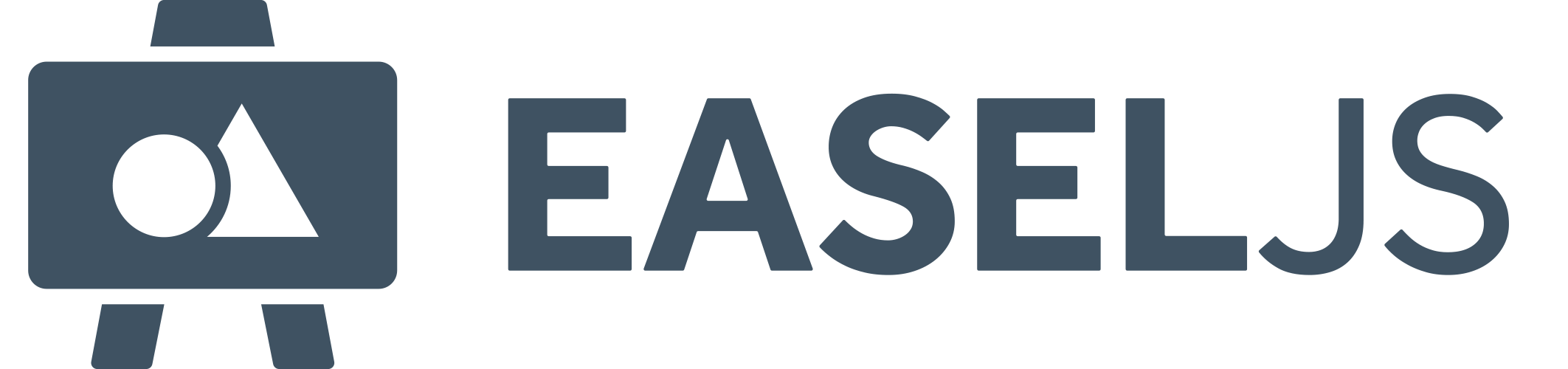#API Documentation for: 1.0.0
Show:

# File:ColorFilter.js

```/*
* ColorFilter
* Visit http://createjs.com/ for documentation, updates and examples.
*
* Copyright (c) 2010 gskinner.com, inc.
*
* Permission is hereby granted, free of charge, to any person
* obtaining a copy of this software and associated documentation
* files (the "Software"), to deal in the Software without
* restriction, including without limitation the rights to use,
* copy, modify, merge, publish, distribute, sublicense, and/or sell
* copies of the Software, and to permit persons to whom the
* Software is furnished to do so, subject to the following
* conditions:
*
* The above copyright notice and this permission notice shall be
* included in all copies or substantial portions of the Software.
*
* THE SOFTWARE IS PROVIDED "AS IS", WITHOUT WARRANTY OF ANY KIND,
* EXPRESS OR IMPLIED, INCLUDING BUT NOT LIMITED TO THE WARRANTIES
* OF MERCHANTABILITY, FITNESS FOR A PARTICULAR PURPOSE AND
* NONINFRINGEMENT. IN NO EVENT SHALL THE AUTHORS OR COPYRIGHT
* HOLDERS BE LIABLE FOR ANY CLAIM, DAMAGES OR OTHER LIABILITY,
* WHETHER IN AN ACTION OF CONTRACT, TORT OR OTHERWISE, ARISING
* FROM, OUT OF OR IN CONNECTION WITH THE SOFTWARE OR THE USE OR
* OTHER DEALINGS IN THE SOFTWARE.
*/

/**
* @module EaselJS
*/

// namespace:
this.createjs = this.createjs||{};

(function() {
"use strict";

// constructor:
/**
* Applies a color transform to DisplayObjects.
*
* <h4>Example</h4>
* This example draws a red circle, and then transforms it to Blue. This is accomplished by multiplying all the channels
* to 0 (except alpha, which is set to 1), and then adding 255 to the blue channel.
*
*      var shape = new createjs.Shape().set({x:100,y:100});
*      shape.graphics.beginFill("#ff0000").drawCircle(0,0,50);
*
*      shape.filters = [
*          new createjs.ColorFilter(0,0,0,1, 0,0,255,0)
*      ];
*      shape.cache(-50, -50, 100, 100);
*
* See {{#crossLink "Filter"}}{{/crossLink}} for an more information on applying filters.
* @class ColorFilter
* @param {Number} [redMultiplier=1] The amount to multiply against the red channel. This is a range between 0 and 1.
* @param {Number} [greenMultiplier=1] The amount to multiply against the green channel. This is a range between 0 and 1.
* @param {Number} [blueMultiplier=1] The amount to multiply against the blue channel. This is a range between 0 and 1.
* @param {Number} [alphaMultiplier=1] The amount to multiply against the alpha channel. This is a range between 0 and 1.
* @param {Number} [redOffset=0] The amount to add to the red channel after it has been multiplied. This is a range
* between -255 and 255.
* @param {Number} [greenOffset=0] The amount to add to the green channel after it has been multiplied. This is a range
* between -255 and 255.
* @param {Number} [blueOffset=0] The amount to add to the blue channel after it has been multiplied. This is a range
* between -255 and 255.
* @param {Number} [alphaOffset=0] The amount to add to the alpha channel after it has been multiplied. This is a range
* between -255 and 255.
* @constructor
* @extends Filter
**/
function ColorFilter(redMultiplier, greenMultiplier, blueMultiplier, alphaMultiplier, redOffset, greenOffset, blueOffset, alphaOffset) {
this.Filter_constructor();

// public properties:
/**
* Red channel multiplier.
* @property redMultiplier
* @type Number
**/
this.redMultiplier = redMultiplier != null ? redMultiplier : 1;

/**
* Green channel multiplier.
* @property greenMultiplier
* @type Number
**/
this.greenMultiplier = greenMultiplier != null ? greenMultiplier : 1;

/**
* Blue channel multiplier.
* @property blueMultiplier
* @type Number
**/
this.blueMultiplier = blueMultiplier != null ? blueMultiplier : 1;

/**
* Alpha channel multiplier.
* @property alphaMultiplier
* @type Number
**/
this.alphaMultiplier = alphaMultiplier != null ? alphaMultiplier : 1;

/**
* Red channel offset (added to value).
* @property redOffset
* @type Number
**/
this.redOffset = redOffset || 0;

/**
* Green channel offset (added to value).
* @property greenOffset
* @type Number
**/
this.greenOffset = greenOffset || 0;

/**
* Blue channel offset (added to value).
* @property blueOffset
* @type Number
**/
this.blueOffset = blueOffset || 0;

/**
* Alpha channel offset (added to value).
* @property alphaOffset
* @type Number
**/
this.alphaOffset = alphaOffset || 0;

this.FRAG_SHADER_BODY = (
"uniform vec4 uColorMultiplier;" +
"uniform vec4 uColorOffset;" +

"void main(void) {" +
"vec4 color = texture2D(uSampler, vRenderCoord);" +

"gl_FragColor = (color * uColorMultiplier) + uColorOffset;" +
"}"
);

}
var p = createjs.extend(ColorFilter, createjs.Filter);

// TODO: deprecated
// p.initialize = function() {}; // searchable for devs wondering where it is. REMOVED. See docs for details.

// public methods:
// Docced in superclass
p.shaderParamSetup = function(gl, stage, shaderProgram) {
gl.uniform4f(
gl.getUniformLocation(shaderProgram, "uColorMultiplier"),
this.redMultiplier, this.greenMultiplier, this.blueMultiplier, this.alphaMultiplier
);

gl.uniform4f(
gl.getUniformLocation(shaderProgram, "uColorOffset"),
this.redOffset/255, this.greenOffset/255, this.blueOffset/255, this.alphaOffset/255
);
};

// Docced in superclass
p.toString = function() {
return "[ColorFilter]";
};

// Docced in superclass
p.clone = function() {
return new ColorFilter(
this.redMultiplier, this.greenMultiplier, this.blueMultiplier, this.alphaMultiplier,
this.redOffset, this.greenOffset, this.blueOffset, this.alphaOffset
);
};

// private methods:
// Docced in superclass
p._applyFilter = function(imageData) {
var data = imageData.data;
var l = data.length;
for (var i=0; i<l; i+=4) {
data[i] = data[i]*this.redMultiplier+this.redOffset;
data[i+1] = data[i+1]*this.greenMultiplier+this.greenOffset;
data[i+2] = data[i+2]*this.blueMultiplier+this.blueOffset;
data[i+3] = data[i+3]*this.alphaMultiplier+this.alphaOffset;
}
return true;
};

createjs.ColorFilter = createjs.promote(ColorFilter, "Filter");
}());

```